# Mathematical problems for self assessment

###### INTEGRATION II

1. By making an appropriate substitution in each case evaluate the integrals:

(i)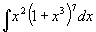(ii)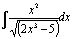(iii)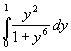[Ans. (i)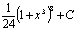(ii)(iii)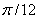]

2. By making the substitution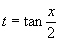evaluate the indefinite integral[Ans.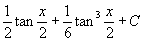]

3. By making the substitution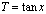evaluate the indefinite integral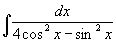[Ans.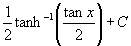or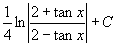]

4. By completing the square evaluate the following integrals

(i)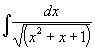(ii)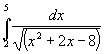[Ans. (i)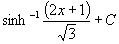(ii) Ans.]

5. Evaluate the indefinite integrals:

(i)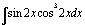(ii)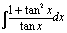[Ans. (i)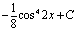(ii)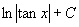]

6. Evaluate the integrals:

(i)(ii)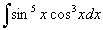(iii)[Ans.(i)(ii)(iii)]

7. Using partial fractions evaluate the definite integrals:

(i)(ii)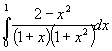[Ans.(i)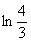(ii)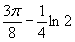]

8. Using partial fractions evaluate the indefinite integral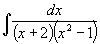[Ans.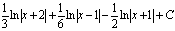]